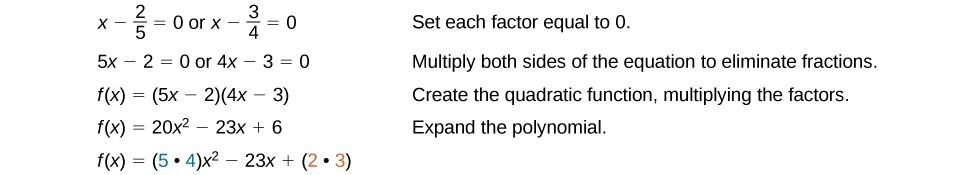## Use the Rational Zero Theorem to find rational zeros

Another use for the Remainder Theorem is to test whether a rational number is a zero for a given polynomial. But first we need a pool of rational numbers to test. The Rational Zero Theorem helps us to narrow down the number of possible rational zeros using the ratio of the factors of the constant term and factors of the leading coefficient of the polynomial

Consider a quadratic function with two zeros, $x=\frac{2}{5}$ and $x=\frac{3}{4}$.

By the Factor Theorem, these zeros have factors associated with them. Let us set each factor equal to 0, and then construct the original quadratic function absent its stretching factor.Notice that two of the factors of the constant term, 6, are the two numerators from the original rational roots: 2 and 3. Similarly, two of the factors from the leading coefficient, 20, are the two denominators from the original rational roots: 5 and 4.

We can infer that the numerators of the rational roots will always be factors of the constant term and the denominators will be factors of the leading coefficient. This is the essence of the Rational Zero Theorem; it is a means to give us a pool of possible rational zeros.

### A General Note: The Rational Zero Theorem

The Rational Zero Theorem states that, if the polynomial $f\left(x\right)={a}_{n}{x}^{n}+{a}_{n - 1}{x}^{n - 1}+…+{a}_{1}x+{a}_{0}$ has integer coefficients, then every rational zero of $f\left(x\right)$ has the form $\frac{p}{q}$ where p is a factor of the constant term ${a}_{0}$ and q is a factor of the leading coefficient ${a}_{n}$.

When the leading coefficient is 1, the possible rational zeros are the factors of the constant term.

### How To: Given a polynomial function $f\left(x\right)$, use the Rational Zero Theorem to find rational zeros.

1. Determine all factors of the constant term and all factors of the leading coefficient.
2. Determine all possible values of $\frac{p}{q}$, where p is a factor of the constant term and q is a factor of the leading coefficient. Be sure to include both positive and negative candidates.
3. Determine which possible zeros are actual zeros by evaluating each case of $f\left(\frac{p}{q}\right)$.

### Example 3: Listing All Possible Rational Zeros

List all possible rational zeros of $f\left(x\right)=2{x}^{4}-5{x}^{3}+{x}^{2}-4$.

### Solution

The only possible rational zeros of $f\left(x\right)$ are the quotients of the factors of the last term, –4, and the factors of the leading coefficient, 2.

The constant term is –4; the factors of –4 are $p=\pm 1,\pm 2,\pm 4$.

The leading coefficient is 2; the factors of 2 are $q=\pm 1,\pm 2$.

If any of the four real zeros are rational zeros, then they will be of one of the following factors of –4 divided by one of the factors of 2.

$\begin{cases}\frac{p}{q}=\pm \frac{1}{1},\pm \frac{1}{2}\text{ }& \frac{p}{q}=\pm \frac{2}{1},\pm \frac{2}{2}\text{ }& \frac{p}{q}=\pm \frac{4}{1},\pm \frac{4}{2}\end{cases}$

Note that $\frac{2}{2}=1$ and $\frac{4}{2}=2$, which have already been listed. So we can shorten our list.

$\frac{p}{q}=\frac{\text{Factors of the last}}{\text{Factors of the first}}=\pm 1,\pm 2,\pm 4,\pm \frac{1}{2}$

### Example 4: Using the Rational Zero Theorem to Find Rational Zeros

Use the Rational Zero Theorem to find the rational zeros of $f\left(x\right)=2{x}^{3}+{x}^{2}-4x+1$.

### Solution

The Rational Zero Theorem tells us that if $\frac{p}{q}$ is a zero of $f\left(x\right)$, then p is a factor of 1 and q is a factor of 2.

$\begin{cases}\frac{p}{q}=\frac{\text{factor of constant term}}{\text{factor of leading coefficient}}\hfill \\ \text{ }=\frac{\text{factor of 1}}{\text{factor of 2}}\hfill \end{cases}$

The factors of 1 are $\pm 1$ and the factors of 2 are $\pm 1$ and $\pm 2$. The possible values for $\frac{p}{q}$ are $\pm 1$ and $\pm \frac{1}{2}$. These are the possible rational zeros for the function. We can determine which of the possible zeros are actual zeros by substituting these values for x in $f\left(x\right)$.

$\begin{cases}\text{ }f\left(-1\right)=2{\left(-1\right)}^{3}+{\left(-1\right)}^{2}-4\left(-1\right)+1=4\hfill \\ \text{ }f\left(1\right)=2{\left(1\right)}^{3}+{\left(1\right)}^{2}-4\left(1\right)+1=0\hfill \\ \text{ }f\left(-\frac{1}{2}\right)=2{\left(-\frac{1}{2}\right)}^{3}+{\left(-\frac{1}{2}\right)}^{2}-4\left(-\frac{1}{2}\right)+1=3\hfill \\ \text{ }f\left(\frac{1}{2}\right)=2{\left(\frac{1}{2}\right)}^{3}+{\left(\frac{1}{2}\right)}^{2}-4\left(\frac{1}{2}\right)+1=-\frac{1}{2}\hfill \end{cases}$

Of those, $-1,-\frac{1}{2},\text{ and }\frac{1}{2}$ are not zeros of $f\left(x\right)$. 1 is the only rational zero of $f\left(x\right)$.

### Try It 3

Use the Rational Zero Theorem to find the rational zeros of $f\left(x\right)={x}^{3}-5{x}^{2}+2x+1$.

Solution